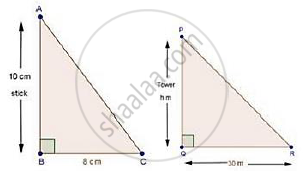Share

# A Vertical Stick 10 Cm Long Casts a Shadow 8 Cm Long. at the Same Time a Shadow 30 M Long. Determine the Height of the Tower. - CBSE Class 10 - Mathematics

ConceptCriteria for Similarity of Triangles

#### Question

A vertical stick 10 cm long casts a shadow 8 cm long. At the same time a shadow 30 m long. Determine the height of the tower.

#### SolutionLength of stick = 10 cm

Length of shadow of stick = 8 cm

Length of shadow of tower = h cm

In ΔABC and ΔPQR

∠B = ∠Q = 90°

And, ∠C = ∠R [Angular elevation of sun]

Then, ΔABC ~ ΔPQR [By AA similarity]

therefore"AB"/"PQ"="BC"/"QR"

rArr"10 cm"/"8 cm"="h cm"/3000

rArrh=10/8xx3000=3750  cm = 37.5 m

Is there an error in this question or solution?

#### Video TutorialsVIEW ALL 

Solution A Vertical Stick 10 Cm Long Casts a Shadow 8 Cm Long. at the Same Time a Shadow 30 M Long. Determine the Height of the Tower. Concept: Criteria for Similarity of Triangles.
S